# Cosmology

### History of the Universe

The average temperature of the universe is inversely related to the scale factor:
temperaturepast / temperaturenow = scale factornow / scale factorpast
= red shift (z) + 1
So as the universe expanded, the temperature dropped. We know that each particle has an energy associated with it through the equation
energy = mass * c2
Similarly, each of the fundamental forces has an energy scale associated with it. By dividing each energy by Boltzmann's constant (1.381 * 10-23 J/K):
temperature freeze out = energy / kB
we find the temperature at which each force becomes effective, and at which each particle condenses from its constituent parts. For instance, above 1015 K, the particles which mediate the weak force (W and Z bosons) are unstable to pair production (decay into an electron and a positron), and interactions which change quark flavors (as in radioactive decay) are not possible. When the temperature drops below that associated with the mass of the proton:
1.673 * 10-27 kg * c2 / kB
= 1013 K
the constituent quarks in the proton are cool enough to be bound together by the strong force:

eventtemperature (K)scale factornow / scale factorthentime
strong forces freeze out10273.7 * 102610-35 s
weak forces freeze out10153.7 * 101410-10 s
protons, neutrons freeze out10133.7 * 10120.0001 s
neutrinos decouple3 * 10101.1 * 10101 s
electrons freeze out6 * 1092.2 * 109100 s
primordial 2H, 4He form9 * 1083.3 * 1082-15 minutes
When the protons and neutrons froze out, the ratio of protons to neutrons was about 6:1 because of the mass difference between the neutron and proton (the neutron is slightly heavier). The large number of neutrons available, as well as the fact that neutron capture occurs faster than proton fusion, caused the nucleosynthesis reactions here to be somewhat different from those taking place in the Sun. Once the universe cooled enough to allow Deuterium (2H) to exist, the ratio was 7:1 (from neutron decays) and the following reactions occurred:
• 1H + n -> 2H + γ
• 2H + 2H -> 3He + n + γ
• 3He + n -> 4He + γ
This sequence lasted about 15 minutes. The final ratio of 4He to 1H was about 1:12, so that the universe was about 75% Hydrogen and 25% Helium by mass. A small amount of Deuterium survived; since it does not survive in stars, what Deuterium we observe is primordial. This is a sensitive indicator of the density of normal matter (not dark) in the universe, since a denser universe would have contained more protons and produced more Deuterium during nucleosynthesis.

Heavier elements were not formed because the temperature and density were both dropping very quickly; in stars they do form because the temperature and density increase (slowly).

We now take up our history:

eventtemperature (K)scale factornow / scale factorthentime
photons decouple, atoms form30001091377000 years
first stars6010.4109 years
today2.7311.378 * 1010 years
Decoupling means that those particles are no longer in thermal equilibrium with their environment. When neutrinos decoupled, the universe became transparent to them; similarly for photons.
The scale factors in the tables above were obtained by using Tnow = 2.725 K, the temperature of the Cosmic Microwave Background Radiation (CMBR). Study of the CMBR has made cosmology an experimental science, as we shall soon see.

The temperatures in our history indicate that the early universe was filled with intense radiation. Even before the decoupling of photons, the density of matter became greater than the density of radiation (due to red-shifting, as we shall see). Wide field surveys indicate that the universe is now very close to being a homogeneous "dust" of galaxy clusters. The pressure from the radiation has all but vanished. But the Type IA supernovae surveys we discussed previously indicate that the expansion of the universe is accelerating. We do not know what causes this acceleration, and for now we simply give it the commonly-used name dark energy. So the universe has passed through three distinct phases:

1. radiation-dominated phase (z > 3250),
2. matter-dominated phase (3250 > z > 0.37), and now
3. dark energy-dominated phase (z < 0.37):

Log-Log plot of temperature vs scale factor.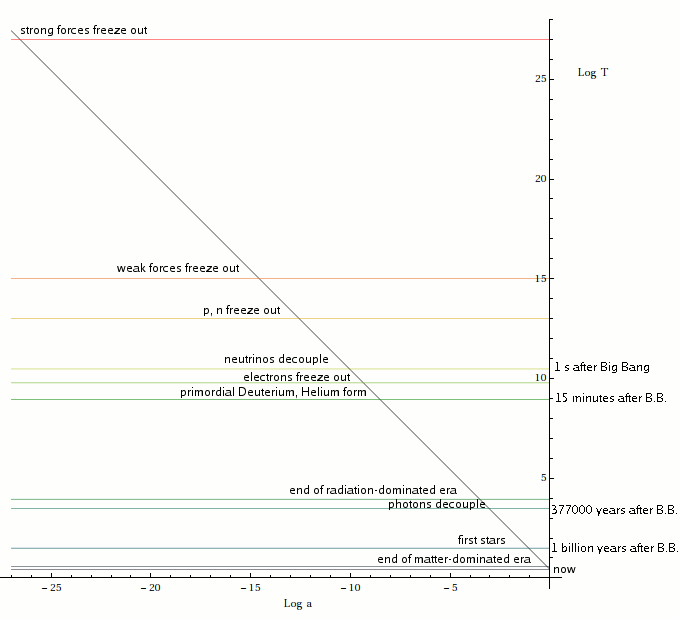### General Relativity

General Relativity (GR), which relates the geometric qualities of spacetime to the matter and energy it is filled with, provides us with a mathematical context for understanding the evolution of the universe. Spacetime is described by a metric: a rule for how to measure intervals. The usual procedure in GR is to find the most general metric which is consistent with the symmetries of the problem at hand, and to find the most general form of the expressions describing the matter and energy. These are related by Einstein's Equations, which are then solved for relations between the free parameters.

Given the wide field surveys and observations of the CMBR, we need the most general metric which is homogeneous and isotropic (the same in all directions). This is the Friedmann-Robertson-Walker (FRW) metric, and it is described by two parameters. The first is "k", the curvature constant; if we choose a time "t" and take all the points in the spacetime which have the same value of t (called a spatial section),

• k = 1 means the section is positively curved:
• cross sections in a fixed direction are circles;
• the universe is closed like a sphere;
• the sum of the interior angles in a triangle is more than 180 degrees;
• k = 0 means the section is not curved; it is flat space:
• cross sections in a fixed direction are straight lines;
• the sum of the interior angles in a triangle is 180 degrees;
• k = -1 means the section is negatively curved:
• cross sections in a fixed direction are hyperbolas;
• the neighborhood of every point in the universe is shaped like a saddle;
• the sum of the interior angles in a triangle is less than 180 degrees:

Surfaces of constant curvature, and their triangles.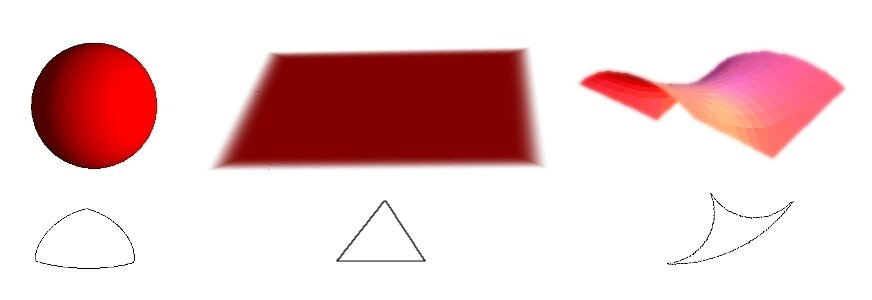(Strictly speaking, what we have said about k, and the images above, are relevant to 2-dimensional surfaces. You have to use your imagination to see how all this applies to 3 dimensions, but with a little practice it can be done.)
For nonpositive k, the universe can be either finite (although obviously very large!) or infinite. A finite universe need not have an edge: a flat closed universe (finite, without boundary) could be like a cube, but with identifications: walking "out" one side is the same as walking "in" the opposite side. Similarly, a negatively curved space can have identifications, but these are much harder to visualize. An infinite universe is called an open universe (without boundary). If k is positive, the universe cannot be open.

The other parameter is a function "a(t)"; this is the scale factor we mentioned earlier. It measures the "size" of the universe as a function of time. The Big Bang occurred when a(t) was zero, and the expansion of the universe means that a(t) increases as a function of time. The Hubble Parameter is defined as the rate of change of a(t) divided by a(t). The rate of change of a(t) is denoted a'(t), and it must be positive as long as the universe is expanding:

H(t) = a'(t) / a(t)
Our matter/energy expression must be able to describe our three act history of the universe: radiation-dominated, matter-dominated and dark energy-dominated. This can be done using a perfect fluid, which is described by its energy density "ρ" and pressure "p" (perfect fluids do not have viscosity or convection). We will usually assume a simple (but reasonable) equation of state (which gives the pressure as a function of the density)
p = w ρ
where "w" is a constant:

• if w=0, the equation describes dust;
• if w=1/3 it describes radiation, and
• if w=-1 it describes the Cosmological Constant.

If the dark energy is not due to a Cosmological Constant, it is usually given the name quintessence, and it has a different equation of state. Current data is consistent with a Cosmological Constant, and we will assume that simple scenario in the following.

With these parameterizations, Einstein's Equations reduce to two simple equations:

a'2(t) (ρ(t) / ρc - 1) = k c2,
and
a''(t) = - (4 π G / 3 c2) a(t) (3 p(t) + ρ(t))
= - (3 w + 1) (4 π G / 3 c2) a(t) ρ(t)
where we have shown explicitly the dependence of the density and pressure on the time (since density and pressure both depend on volume and therefore on the scale factor, which is a function of time). ρc is the critical density
3 H2 c2 / 8 π G
= 8.2487 * 10-10 J / m3,
equivalent to about 5.5 protons per cubic meter.

• If ρ = ρc, k must equal zero.
• If ρ > ρc, k must be positive, and
• if ρ < ρc, k must be negative.
Note that unless w < -1/3, the second of Einstein's Equations implies that the acceleration of the universe must slow down (since a''(t)<0).

Einstein's Equations are supplemented by a conservation equation which guarantees that energy is conserved. With our simple equation of state, the conservation equation has the solution

ρ(t) is proportional to 1 / a3(w + 1)(t).
This tells us something we already knew: for matter, the density drops as the volume increases, and the radiation pressure drops as a4(t) increases, due to the combination of the increase in volume and the red shift.
Note that for the Cosmological Constant, w = -1, so ρ is a constant: it does not depend on a or t.
It is customary to use the ratio ρ / ρc as a cosmological parameter. This quantity is denoted "Ω". Using it, our first Einstein Equation (also called the Friedmann Equation) is written
a'2(t) (Ω - 1) = k c2
So if Ω = 1, k must be zero; if Ω < 1, k must equal -1, and if Ω > 1, k must equal 1.

When the universe cooled so much that there was insufficient energy to ionize Hydrogen atoms, the universe became transparent to photons. Before then, it consisted of a dense plasma (electrically charged fluid) containing electrons, baryons, and photons. There was also dark matter: massive particles (not yet understood as part of the Standard Model) which do not exchange photons, and so do not interact electromagnetically. Dark matter may come in two forms: Cold Dark Matter (CDM) or Hot Dark Matter (HDM); HDM travels at speeds close to that of light, while CDM is non-relativistic (speeds ≤ 50 km/s). There was also dark energy, but its influence appears to have been negligible in the early universe.

• Since the baryons are roughly 2000 times more massive than electrons, they were the fundamental source of inertia (resistance to acceleration).
• Baryons and dark matter were the fundamental sources of gravitational attraction.
• Photons were the fundamental source of pressure, which was therefore experienced by the electrons and baryons but not the dark matter.
• Neutrinos contributed to the radiation energy density because they are nearly massless and move at relativistic speeds, but because they are electrically neutral they did not contribute to the pressure: they simply passed through the plasma unimpeded.

Because of their differing physical effects, the contributions of radiation, baryons, HDM, CDM and the dark energy to Ω are distinguished by subscripts:

Ω = Ωrad + Ωb + ΩHDM + ΩCDM + Ωvac
where "vac" denotes the dark energy contribution. Sometimes "Λ" is used instead of "vac" when we are particularly interested in the cosmological constant. In addition, we sometimes write
ΩM = Ωb + ΩHDM + ΩCDM
to denote the contribution of matter to Ω.

### Solving the Friedmann Equation

With a choice of k and w, we can solve the Friedmann Equation for radiation, matter or a Cosmological Constant:

Solutions of the Friedmann Equation for radiation (in red), dust (in green) and Cosmological Constant (in blue), for each value of k.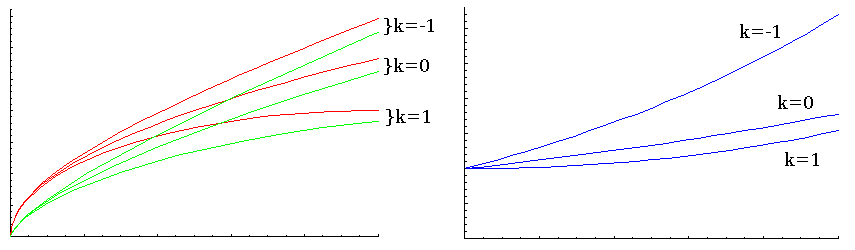The graph on the right shows the solutions for a Cosmological Constant; these solutions are exponential and must be fitted to the appropriate solution from the graph on the left at the appropriate time. From these graphs we see that for smaller values of k, the universe expands more rapidly, and that radiation tends to make the universe expand more rapidly than dust.

The universe of course has all three components: matter, radiation and Cosmological Constant. When all three are present, it is quite difficult to solve the Friedmann Equation analytically, but it can be solved numerically. In order to do so, we must have values for the various Ωs and k.

While our equation of state above implies that we can have either matter or radiation, but not both, the solutions to the conservation equation are valid even when both are present. Equating the energy densities as functions of the scale factor allows us to find the red shift at the transition from the radiation-dominated era to the matter-dominated era:

Using ΩM = 0.278, we can invert the equation to compute Ωrad = 8.55 * 10-5, which includes contributions from both photons and neutrinos.
The energy density from photons alone can be found from the black body distribution as
ργ = 8 π5 (kB * TCMBR)4 / (15 (h c)3)
= 5.06 * 10-5 ρc
We can perform a similar calculation, using ρΛ ≡ ρmatter, to find Ω&Lambda.

Portfolio Exercise: Verify the computations of Ωrad and ργ, and compute Ω&Lambda. For Ω&Lambda, use zM→Λ = 0.374397.

For our numerical solution, we have assumed values for H0, zrad→M, ΩM, ΩΛ and k consistent with the WMAP7+BAO+SNSALT data set for the ΛCDM model. The numerical solution for the scale factor is shown below in blue; the left plot shows the past, and the right plot shows the future:

The scale factor up to the present (left) and in the future (right).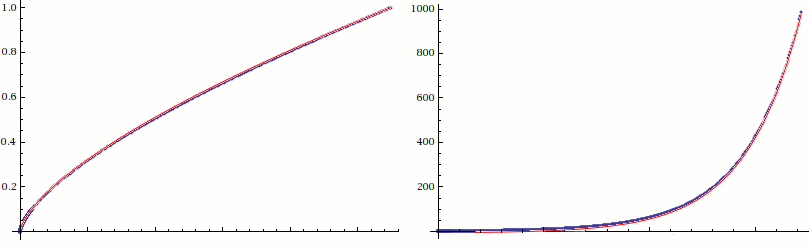Up to the present day, the numerical scale factor is approximately

apast(t) = (t / t now)2/3
(left, in red), but in the distant future it increases exponentially:
afuture(t) = 4/3 ec t √ (Λ / 3)
(right, in red). apast(t) and afuture(t) are the solutions for the matter-dominated era and the Cosmological Constant-dominated eras, respectively. Since they are in such good agreement with the complete numerical solution, we will use them in the following. Of course they do not quite match up, and they neglect the fact that in the recent past, a''(t) becomes positive, as it must since we observe the expansion of the universe to be accelerating. For those shortcomings, they still are very good approximations, and will illustrate all of the qualitative points we wish to make.
There's a caveat here: for the past, we are using the solution for the matter-dominated universe, and in the early stages of its evolution, the universe was dominated by radiation. The scale factor was essentially linear in t during that era, but we can't match the solutions easily because we have no distance information from those early times. So we will pretend this value is accurate, but remember that this is only an approximation.
Recalling our discussion of the Hubble relation, we know that the expansion speed of an object located at a distance r(t) is
vexp = H(t) * r(t)
= a'(t) * r(t) / a(t)

= a'(t) * r(tnow),

since r(t) = r(tnow) * a(t). Using apast(t) for a(t), we see that the Hubble parameter changes with time up to the present as
H(a) = 2 / (3 tnow a3/2)
The Hubble parameter / H0, from past to present as a function of scale factor.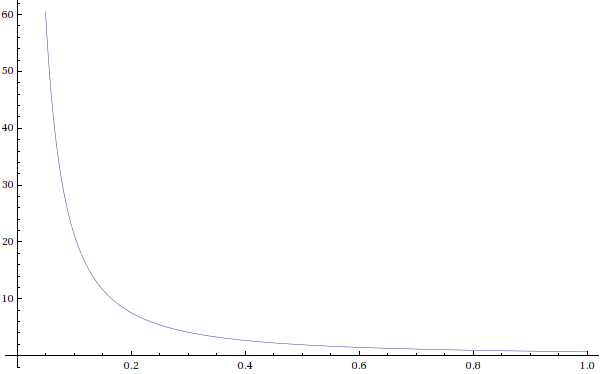But using a(t) = afuture(t) implies that in the future, the Hubble parameter approaches a constant: c √ (Λ/3), or approximately 11.85 km/s / Mpc.

We have seen images of proto-galaxies at z = 1 and beyond. Let us assume that an extraterrestrial named Bob lives on an early-developed planet in one of those protogalaxies (at z=1), and is not moving with respect to it. Using apast(t), it is possible to compute the current distance to Bob, even though the light he emits now will not be seen by us for a long time. Our equation for vexp is actually a differential equation:

dr(t)/dt = a'(t)/a(t) * r(t)
If we consider a light ray traveling toward us from the past, this equation describes the path of the ray as affected by the expansion of the universe:
dr(t)/dt = a'past(t)/apast(t) * r(t) - c
The expansion of the universe essentially modifies the speed of light, which is only guaranteed to be constant in inertial frames: coordinate systems moving at constant velocity. The solution to this equation is
rpast(t) = bpast * t2/3 - 3 c t,
where bpast is a constant to be determined by setting rpast(tnow) = 0, since we are measuring distances relative to our current position (where we see the light from Bob).

Since Bob was at z = 1 when he emitted light that we are just seeing now at z = 0 (a = 1), we can find the time since the emission by setting

apast(tthen) = 1 / (z + 1)
and solving for tthen. Using the current age of the universe for tnow we can find bpast, and therefore Bob's distance when he emitted the light (rpast(tthen)). By multiplying by anow/apast, we then obtain his current distance rnow from us. We find that Bob is currently 1.146 * 1026, or 3.71 Gpc, away.

With Bob's current distance from us, we can now compute Bob's distance from us at any time, and his recession velocity at any time as well. Here are plots of his recession velocity (with c = 1), in the past (on the left) and in the future (on the right). The horizontal scale is the scale factor, and the red line corresponds to Bob, while the blue line corresponds to an object midway between us:

Expansion speeds of Bob (red) and an object between us (blue), vs scale factor (c = 1).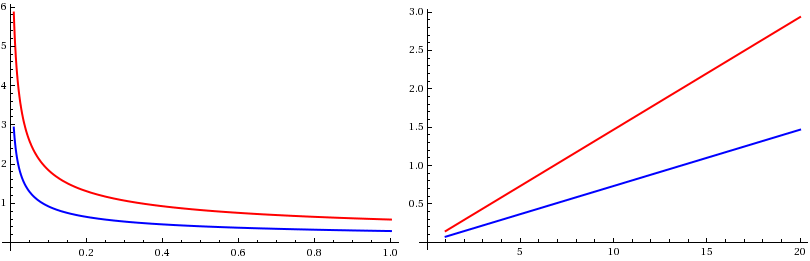We can see that Bob's recession velocity is greater than the speed of light for a < 0.26 (in the past) and a > 6.8 (in the future). We also see that the recession velocity of objects closer than Bob dropped below the speed of light earlier than Bob's did, and will become greater than the speed of light later than Bob's will. The opposite is true of objects further from us than Bob; the length of time an object's recession velocity is below the speed of light varies inversely with its distance from us.

None of this contradicts what we have said previously about nothing being able to move faster than the speed of light. Bob is at rest: his velocity relative to his neighbors is zero. The distance between events is increasing faster than the speed of light because the universe is expanding there at that rate.
If we plot Bob's position at various times in his history (he is a very long-lived extraterrestrial), we see that before the scale factor was 0.26 (left), his world line is spacelike, and after that it is timelike. It becomes spacelike again after the scale factor becomes greater than 6.8 (right):

r(t) for Bob; the black line is the edge of the light cone (past on the left, future on the right).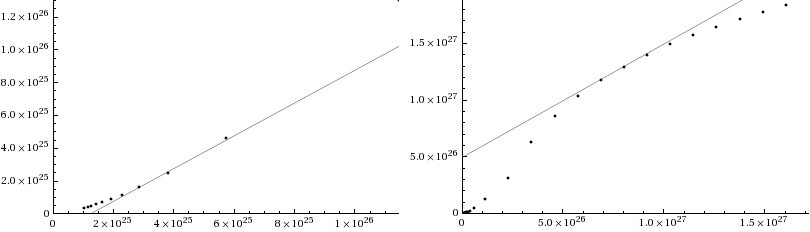Once we have rnow, we can find the path of a light ray from Bob at any time by setting rpast(tthen) equal to rnow*athen, and solving for a new bpast. There will be a new bpast for every light ray emitted by Bob at a different time.

If we consider a light ray traveling toward us from Bob at some time in the future, the solution is

rfuture(t) = bfuture * ec t √ (Λ / 3) + √ (3 / &Lambda),
where bfuture is a different constant to be determined by setting rfuture(tthen) = rnow*athen. (To find tthen, we set afuture(tthen) equal to athen and solve for tthen.)
These computations help us to realize what it means to study General Relativity!
Bob's light cones are defined by a "left edge" and a "right edge". The left edge is just rpast(t) (or rfuture(t)) that we found above. The right edge is found using
dr(t)/dt = a'past(t)/apast(t) * r(t) + c,
with new solutions
rpast(t) = bpast * t2/3 + 3 c t
and
rfuture(t) = bfuture * ec t √ (Λ / 3) - √ (3 / &Lambda).
Note that these equations have different values for bpast and bfuture, but those values are found the same way as before, since both "edges" of the light cone start at the same point.

We can solve these equations for the light rays passing through any event on Bob's world line, and so reconstruct his light cones at several interesting times: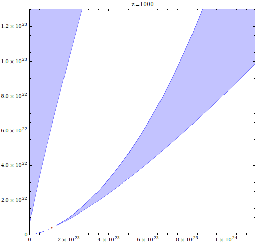Light cones for Bob when the scale factor was 1/1001; was there a common past?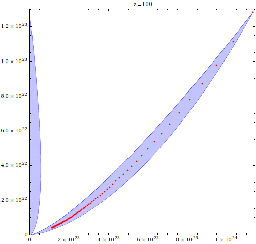Light cones for Bob when the scale factor was 1/101.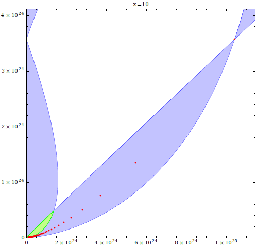Light cones for Bob when the scale factor was 1/11: Bob can see our early history.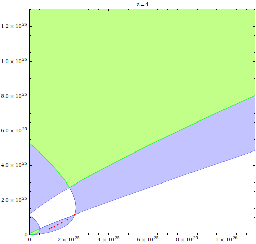Light cones for Bob when the scale factor was 1/5; Bob's recession speed is still > c.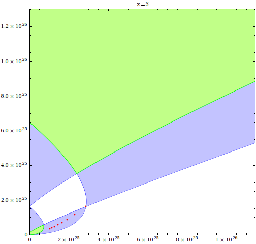Light cones for Bob when the scale factor was 1/4; Bob's recession speed is about to drop below c.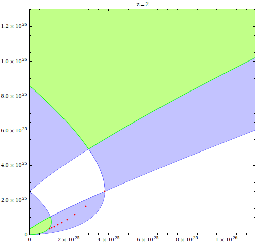Light cones for Bob when the scale factor was 1/3; Bob's recession speed is < c.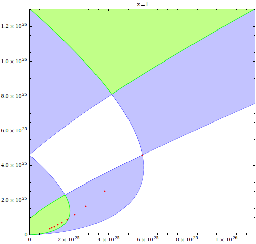Light cones for Bob (scale factor = 1/2); we can now see his protogalaxy.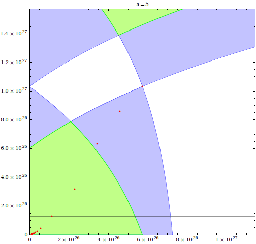Light cones for Bob when the scale factor will be 5; Bob's recession speed is still < c.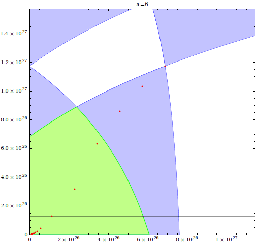Light cones for Bob when the scale factor will be 6; Bob's recession speed is about to go superluminal.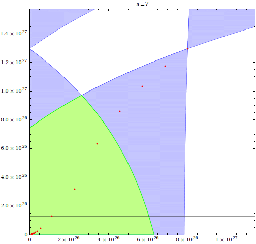Light cones for Bob when the scale factor will be 7; Bob's recession speed is > c.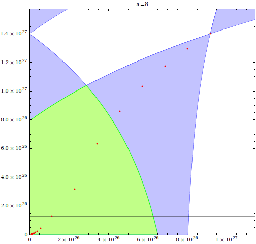Light cones for Bob when the scale factor will be 8.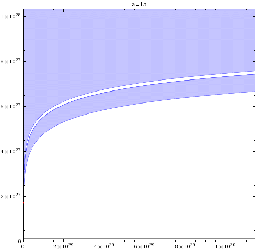Light cones for Bob when the scale factor will be 15; Bob will never see us again, and vice versa.

Notice first that these light cones are not at 45 degree angles: they are warped by the expansion of the universe, whose expansion speeds depend on position. The red dots outline Bob's world line, and the ends of the axes correspond to a = 1/101, a = 1/11, a = 1 and a = 10 (except for the last plot). The horizontal black line on the future plots (a > 0) represents the present.

As we discussed previously, the blue area below an event on a world line is the past light cone of that event, and the blue area above it is its future light cone. The world line of events taking place at the origin is simply the vertical axis, on the left edge of each frame. The green regions are where light cones from our world line and Bob's overlap. A green region below an event indicates a point in our mutual causal past: a prior event there is visible from the two later events. A green region above an event indicates a point in our mutual causal future: an event at one will eventually be visible there. The horizontal axis represents the time of the Big Bang; at that time, the entire universe is the point at the origin.

It appears that Bob's events at a = 1/1001 are causally disconnected from those at the origin. But we must be cautious about over-interpreting this graph; the resolution is insufficient to determine whether the light cones overlap at very early times. However, by a = 1/11 it is clear that events at both locations are causally connected: they have a common past.

Just after a = 1/4, the incoming light ray from Bob tilts to the left of vertical: Bob's recession speed has dropped below that of light. Because we see Bob currently at a = 1/2, that plot shows the incoming ray arriving at the origin at the top of the frame: the present. Just before a = 7, the incoming light ray from Bob tilts back to the right of vertical as Bob is again receeding from us faster than light. But by the time the universe is 15 times its current size, Bob's light cone will no longer share a future with ours.

In general, the further away something is, the later we first see it, and the sooner it will move beyond our particle horizon. We do not know if anything exists outside our current particle horizon, but depending on how large the universe actually is, there may be portions that we will never see. Of course nothing is "visible" until after a = 1/11: before then, there were no stars, and the universe was filled with an infrared glow that eventually red shifted to become the CMBR.

Putting all this together, we see the universe expanding so rapidly during its early history that more distant regions evolved independently. In the future, the accelerating expansion will move the galactic clusters out of each others' future light cones, and each will eventually grow cold surrounded only by the void of the then much colder CMBR. This of course will not happen until the universe is many times older than it is now.

Perhaps we should take some consolation in realizing that we live at a point in the life of the universe when it is possible to understand its evolution. In the far future, these notions would not ever be testable; much less would it occur to us to test them.

#### A Small Detail...

These graphs place us at rest at the origin; but what do we mean by the word "origin" if the universe is homogeneous and isotropic? If the universe looks the same from every position, where is the center?
When we solved Einstein's Equations, we used co-moving coordinates: coordinates which move with the point in question. So in our solution, any point in the universe could just as well be the origin, and the horizontal distance in the light-cone diagrams is simply the separation between two points which are "riding along" with the expansion of spacetime.
Note that it is only on the scale of clusters of galaxies that the universe appears homogeneous and isotropic; clearly, the universe looks different to us on Earth than it does if we were, say, on the moon. So when we say that spacetime is expanding, we mean that the distances between galactic clusters are growing with the scale factor, not that the distance between your nose and your eyes is changing. It might be, but the Friedmann Equation has nothing to say about that: it simply does not apply to scales smaller than megaparsecs. If the distance between your nose and your eyes were changing, and it is, for example, 1 cm now, the current value of the Hubble Parameter tells us that the rate of separation would be
1 cm * 69.9 km/s / Mpc
= 10-2 m * 2.265 * 10-18 / s

= 2.265 * 10-20 m/s,

or about 1/100,000th of the diameter of an atomic nucleus each second. In your lifetime, the expansion of the universe would change that distance about the radius of an atom. So even if the Friedmann Equation did imply that the distance between atoms is changing with the expansion of the universe (which it does not!), it would be undetectable on such small scales.